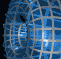Português | English Beyond 3D > Necklaces > z-Cubed Necklace > Movies

# Movies of the z-Cubed NecklaceThis movie shows the complete sequence of rotations from which the five in the "z-Cubed Necklace" were taken. Notice that the projection into two dimensions maintains the four-fold symmetry throughout as the image moves from a disk to a triply covered disk containing a ramification point.

` `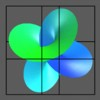This movie shows the complete sequence of rotations from which the five in the "z-Cubed Necklace" were taken. Here the surface is enclosed in a bounding hyper-box to make the four-dimensional rotations easier to follow.

` `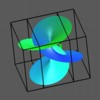This movie shows the same sequence as the previous movie, but from a different viewpoint in space, one where the symmetry is less apparent. The rotation in four-space is a composition of two rotations, one in the xw-plane and one in the yz-plane. These can be seen in this movie as the "sliding box" rotation in the hypercube (due to the xw rotation) and a rotation in three-space (from the yz rotation).

` `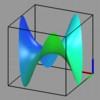The five views in "z-Cubed Necklace" are projections of the complex cubing function into three-space. We can get a better idea of the shape of the projection by looking at a sequence of three-dimensional images. Here we see the first projection as a three-dimensional object. From above it looks like a disk, but it is actually a monkey saddle (the real part of the cube).

` `This movie shows the second projection from "z-Cubed Necklace" rotating in three-space. Opposite ends of the saddle are beginning to squeeze shut as other parts expand.

` `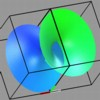This is the third projection of the complex squaring function, projected into three-space, then rotated in space. In this projection, the surface has begin to intersect itself.

` `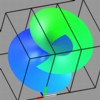This fourth projection of the cubing function shows the surface as it continues to pass through itself, rotated in three-space.

` `This final movie shows the last view of the surface, which we can think of as the graph of the imaginary part of the inverse of the cube (i.e., the complex cubed root). Here, the ramification point appears as a pinch point on the surface, at the origin. The self-intersection appears as a cubic curve on the surface.

` `

See the description of movie formats and plug-ins
if you are having difficulty getting these movies to play.

```
```